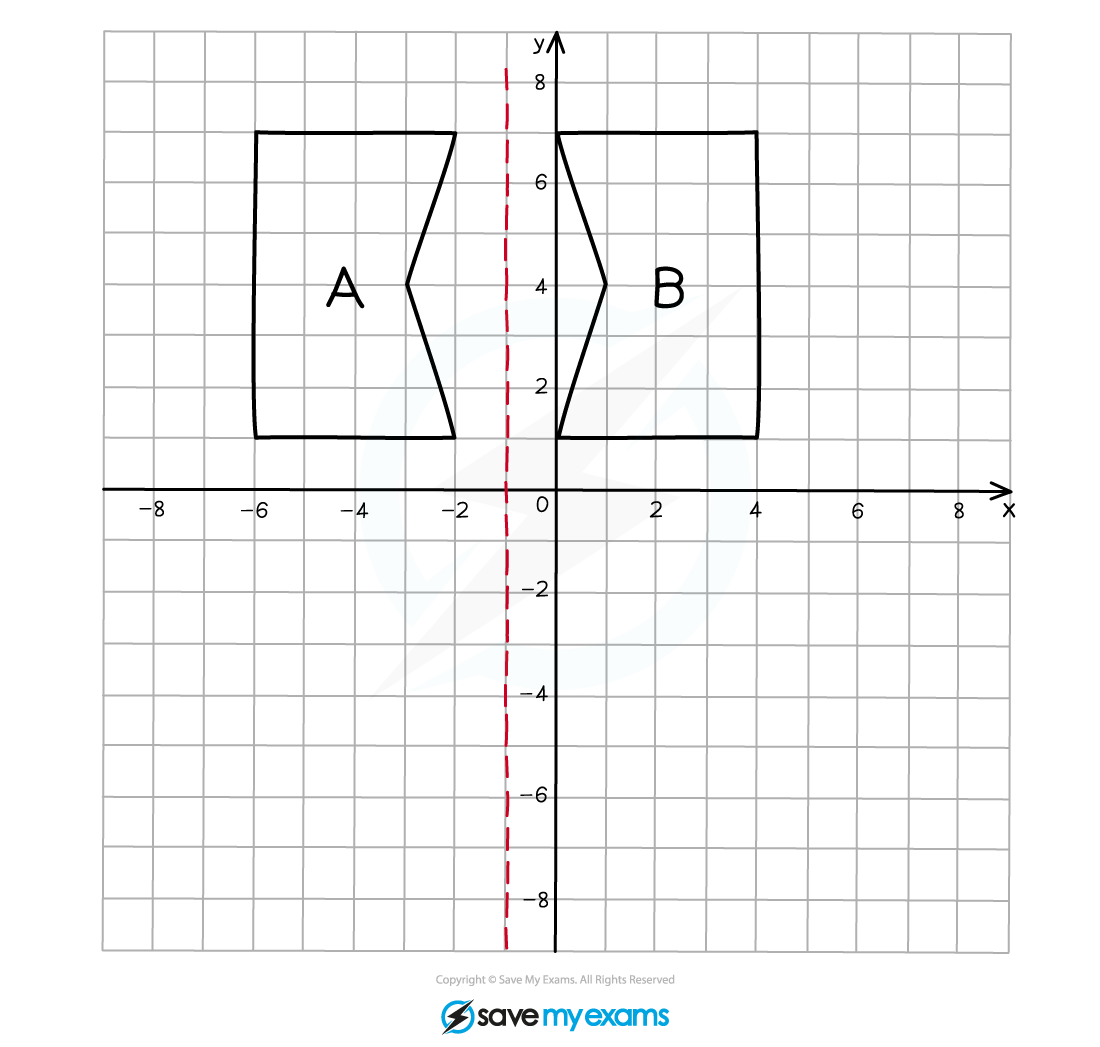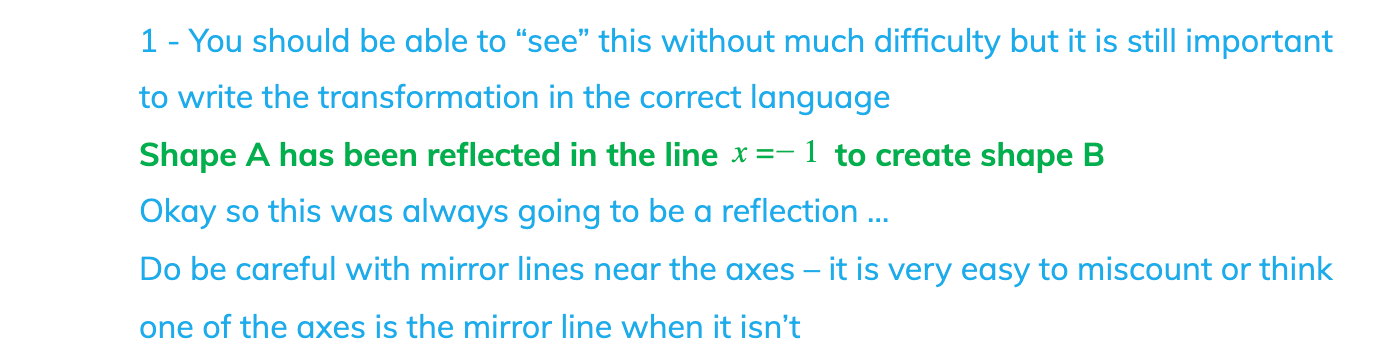# Edexcel IGCSE Maths 复习笔记 5.2.2 Transformations - Reflection

Edexcel IGCSE Maths 复习笔记 5.2.2 Transformations - Reflection

#### Reflection – what do I need to know?

• You need to be able to perform a reflection (on a coordinate grid) as well as spotting and describing a reflection when presented with one
• Reflections only have one key thing to look for – the mirror line – but these will be described mathematically using the equations of straight lines making things a little more awkward

1. Vertical lines

These are in the form x = k, for some number k

2. Horizontal lines

These are in the form y = k, for some number k

3. Diagonal lines

Much harder to perform a reflection in these but lines are of the form

y = mx + c (see Straight Line Graphs)

4. Points on the mirror line

Do not move – they stay where they are

5. Double reflections

This is where the mirror line passes through the shape being reflected

Part of the shape gets reflected one way, the rest the other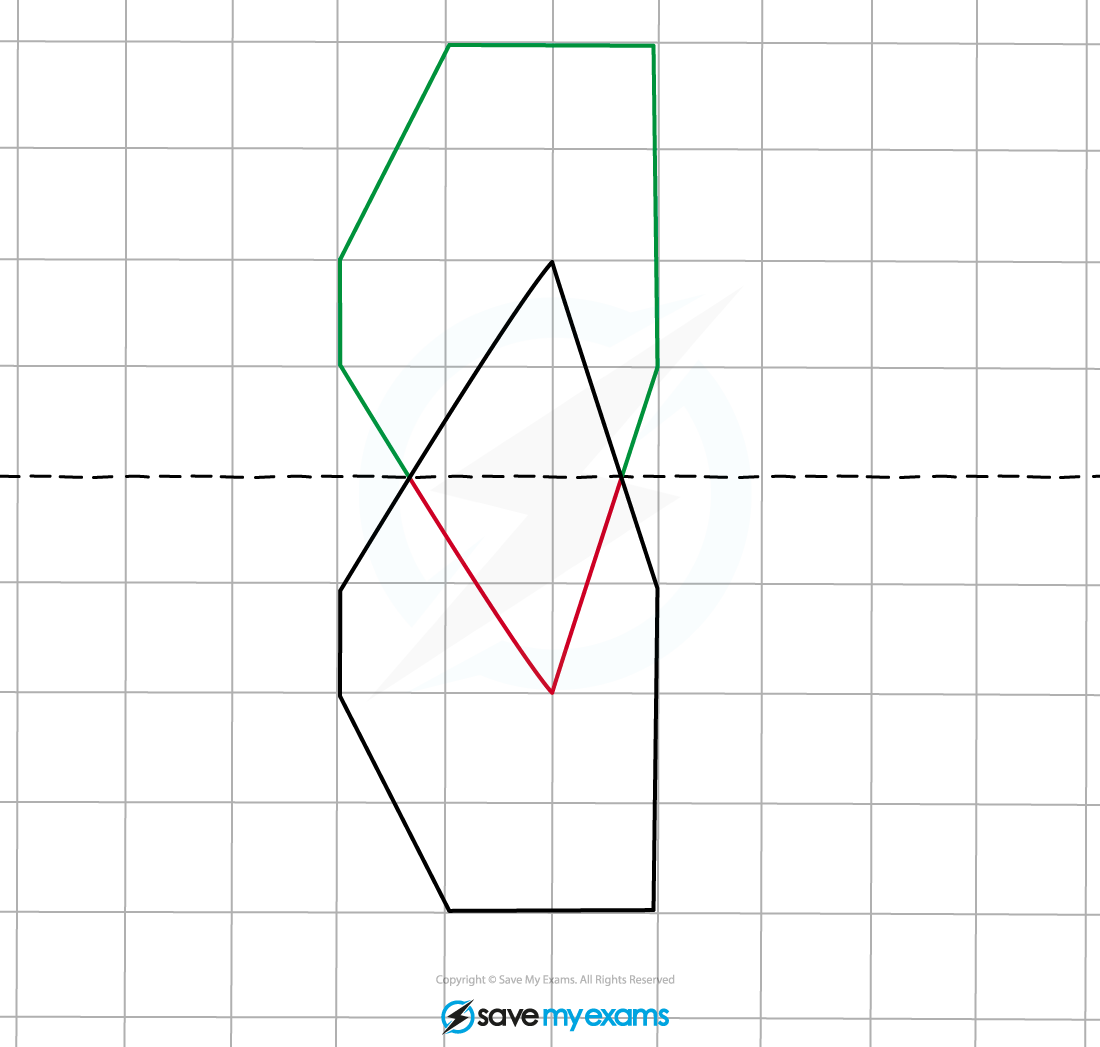6. Regular polygons

Squares and other regular polygons can look identical even after a reflection (and other transformations too) – there is no obvious sign the shape has been reflected – you may think a shape has been translated

The way to identify these is to look at one vertex (point) on the shape and its corresponding position

If it is a reflection it will be “back-to-front” on the other side#### Worked Example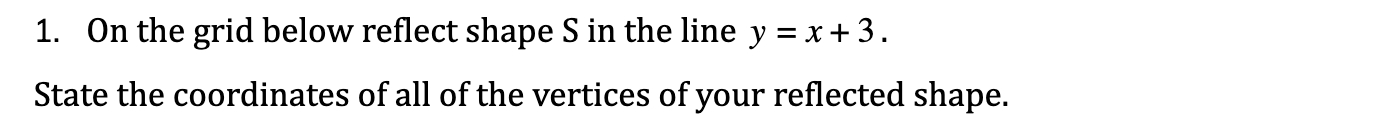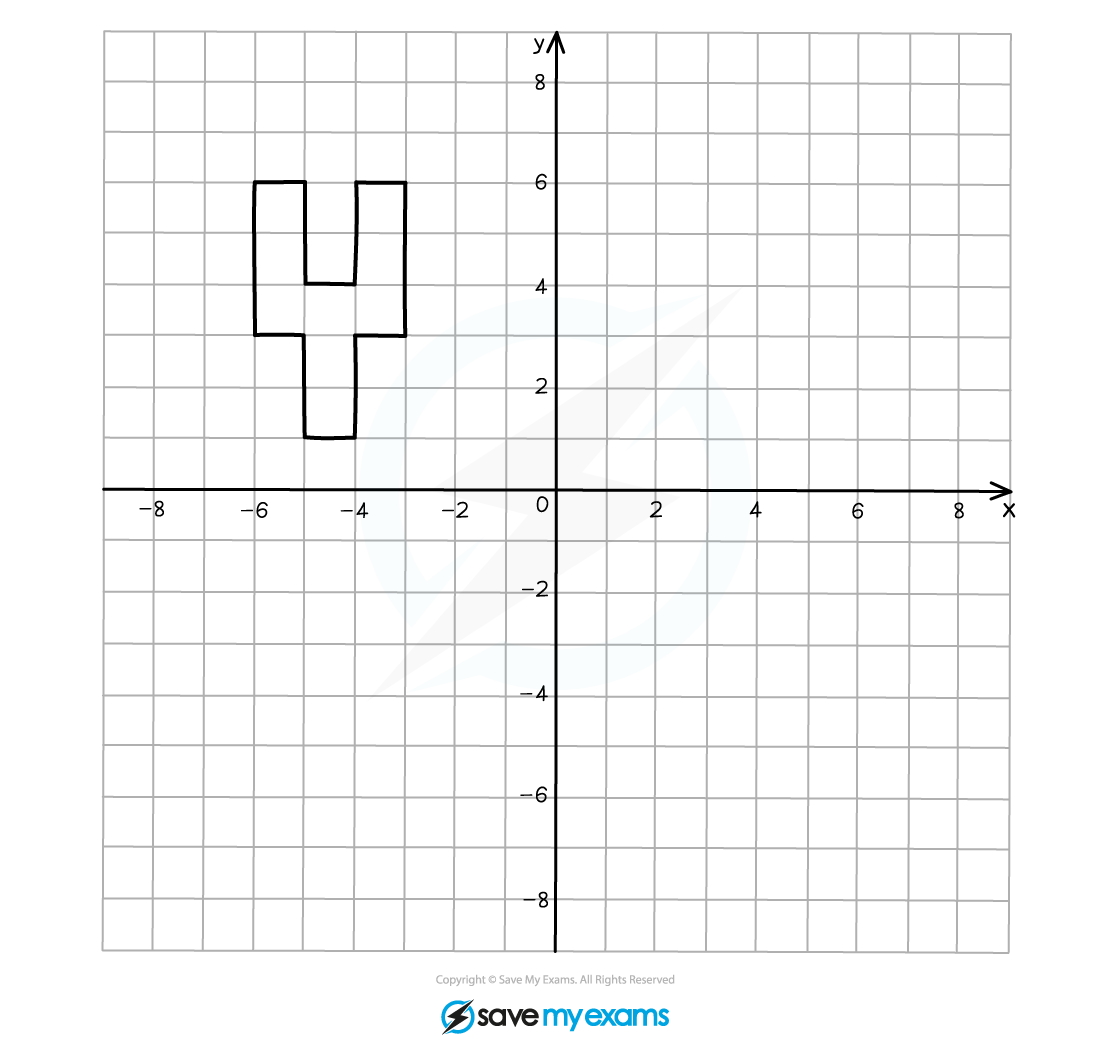#### Worked Example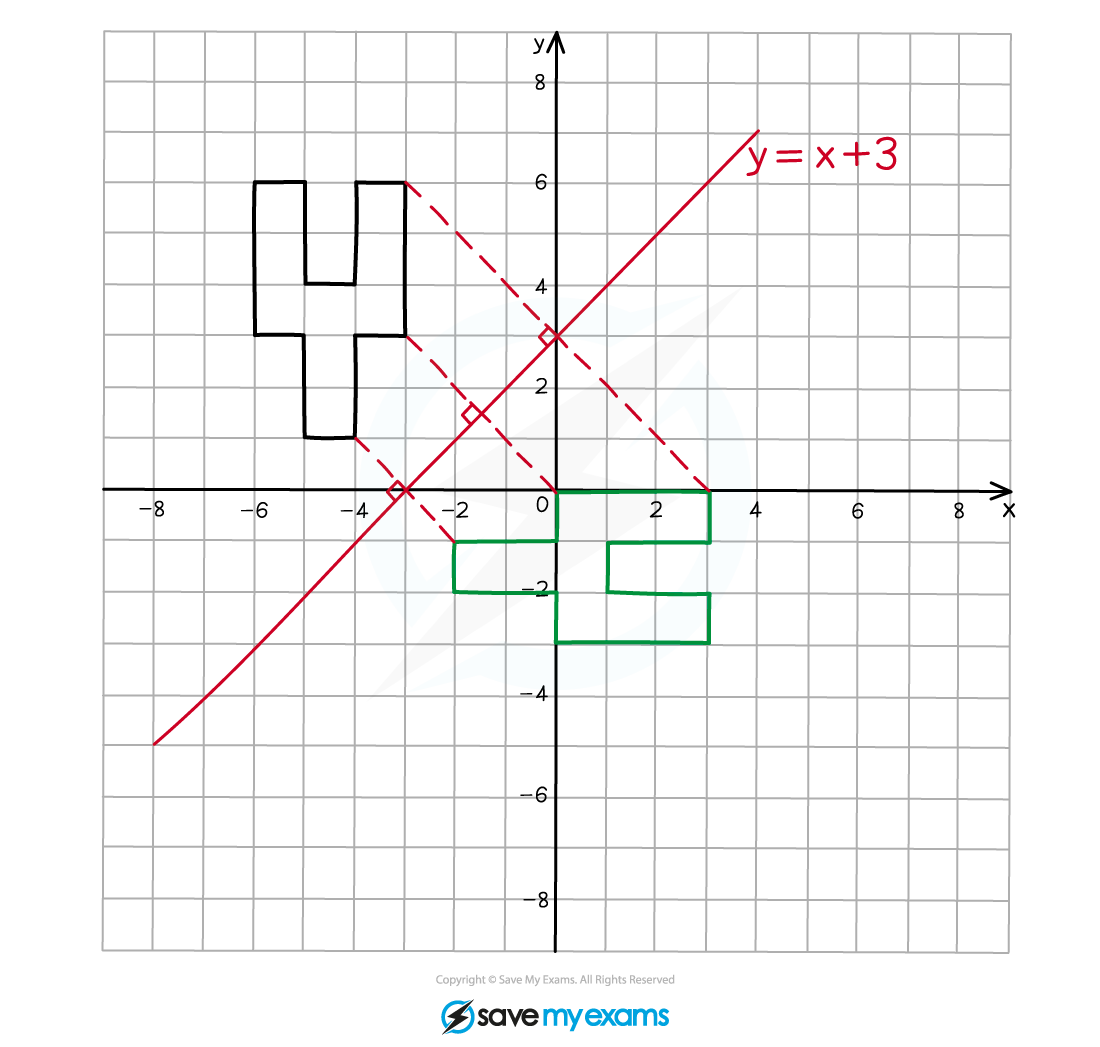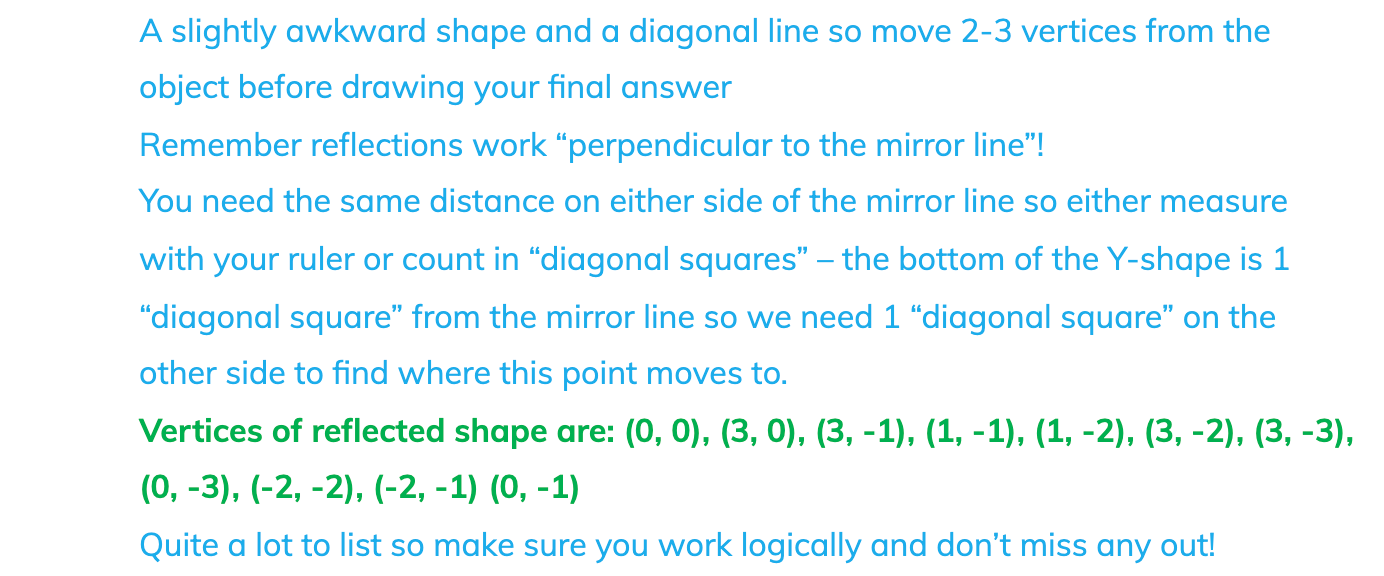#### Worked Example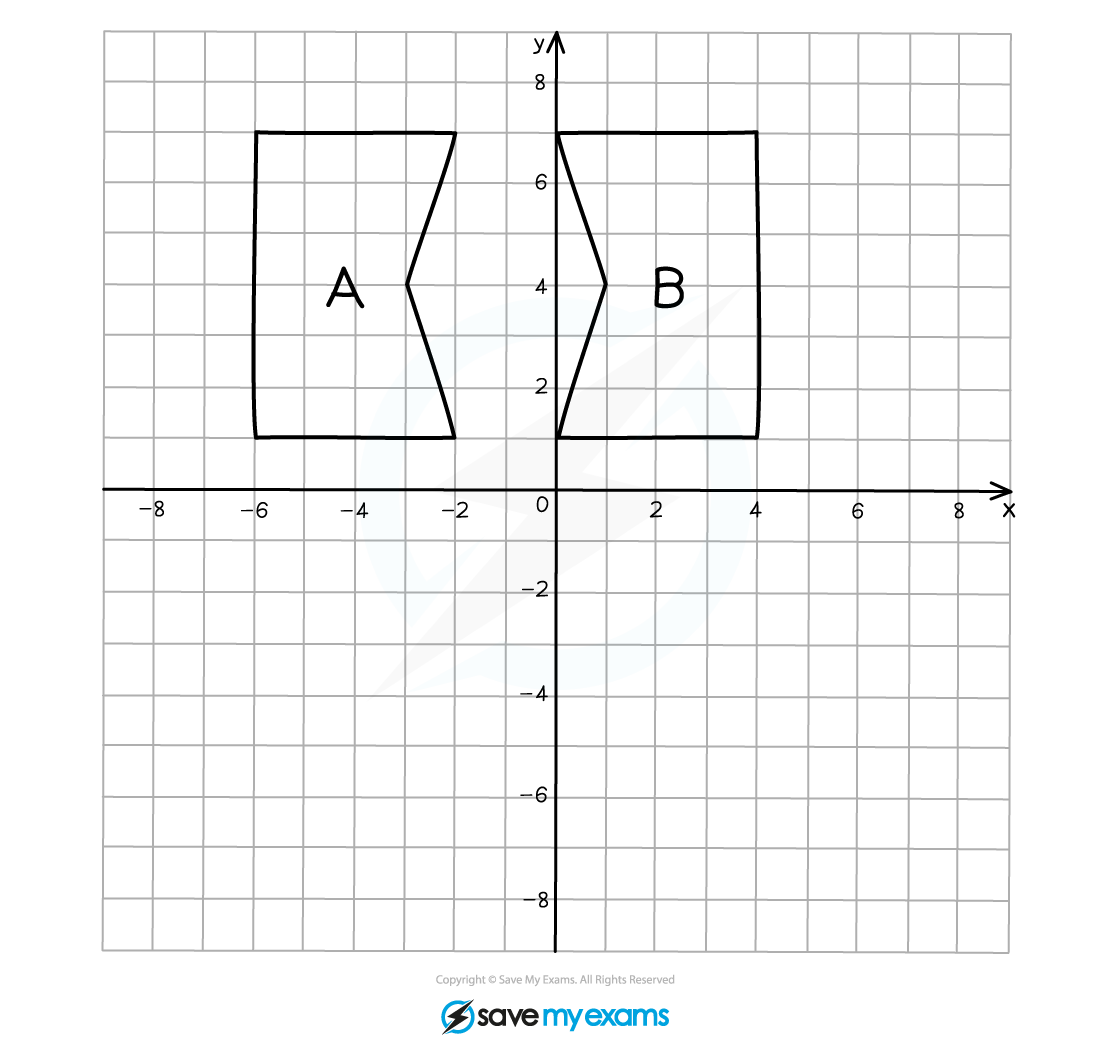#### Worked Example